Disclosure: This post may contain affiliate links, meaning when you click the links and make a purchase, we receive a commission.

# How to Make a Frequency Distribution Table & Graph in Excel?

You can make a histogram or frequency distribution table in Excel in a good number of ways. I have summarized total 7 methods in this article.

In addition, I have created an Excel Template [I named it FreqGen] to make frequency distribution table automatically. Just input data in the template and get frequency distribution table automatically.

Except for these 7 methods, if you know any other technique, let me know in the comment section.

## Terminology of a Frequency Distribution Table

Before going into the discussion of how to make a frequency distribution table in Excel, at first I want to introduce you to the terminology of frequency distribution table.

Look at the following numbers. These are the math scores of 20 students in an exam.

40, 43, 54, 62, 88, 31, 94, 83, 81, 75, 62, 53, 62, 83, 90, 67, 58, 100, 74, 59

Just think yourself as the teacher of these students.

Learn Excel Online: Top Excel Courses Online

 Numerical Scores Letter Grade >=80 A 70-79 A- 60-69 B 50-59 C 40-49 D <40 F

Your task is to categorize the above scores to find out –

1. How many students got A
2. How many students got A-
3. How many students got B
4. How many students got C
5. How many students got D
6. And how many students failed (grade F) in the exam.

Read More: How to Use Compound Interest Formula in Excel

As the number of students is only 20, you can make a frequency distribution table manually without using any formula or sophisticated tool (for example Pivot Table) in Excel. But if you are a statistician or work with big data, you might have to deal with thousands of numbers if not millions of numbers. And one thing is sure: you cannot avoid the errors that might arise from manual process.

In the following image, you see I have made a frequency distribution table. I did it manually and it is just to introduce you to the terms related to a frequency distribution table.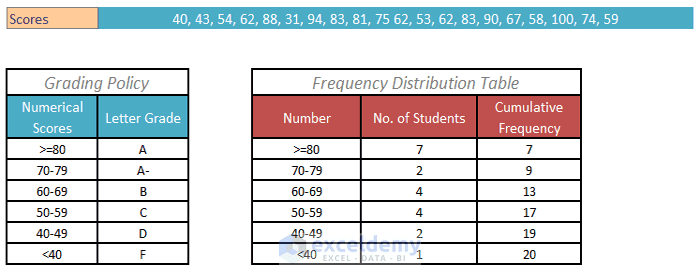A frequency distribution table.

• Bin: In the above image, there are 6 bins. They are >=80, 70-79, 60-69, 50-59, 40-49, and < 40.
• Bin Size: The size of the first bin (>=80) is 21. As from 80 to 100, there are 21 numbers. The size of the second bin (70-79), third bin (60-69), fourth bin (50-59), and fifth bin (40-49) is 10 as there are 10 numbers in every bin. The size of the last bin (<40) is 40 as from 0 to 39 there are 40 values.
• Frequency: Frequency is how many values are counted for a bin. For example for bin 70-79 we have found 2 scores. So the frequency of bin 70-79 is 2. For bin 50-59 we have found 4 scores. So the frequency of bin 50-59 is 4.
• Cumulative Frequency: You get the cumulative frequency from standard frequency. On the above image you see there is a Cumulative Frequency column. The first frequency is 7, it is same as the standard frequency 7 on the left. Next cumulative frequency is 9. 9 is found summing standard frequency 7 and 2 (7+2=9). In the same way, you can find next cumulative frequency 13 (7+2+4), next one 17 ( 7+2+4+4), next cumulative frequency 19 ( 7+2+4+4+2) and the last one 20 ( 7+2+4+4+2+1).

So, you now know the terminologies related to a frequency distribution table.

## Prepare Your Data at First

Before you make a frequency distribution table in Excel, you have to prepare your data in the following ways:

1. At first find out the lowest and highest value from your data set. You can use Excel MIN () and MAX () functions to find out the lowest and highest value respectively. Or you can use Excel features: Sort Smallest to Largest, Sort Largest to Smallest or Sort to sort data and then find out the smallest and largest values from a data set. I prefer you to use MIN () and MAX ().  These two will not change your data arrangement.
2. Then decide how many bins you want to create. It is better to keep your number of bins between 5 and 15. 10 bins is ideal.
3. Bin size will depend on how many bins you want to create. Say the lowest value is 23 and highest value is 252. And you want to make 10 bins. Your bin size will be: (Highest value – Lowest value)/Bin Size = (252-23)/10=22.9. 22.9 or 23 is not a good bin size. I make it 25.
4. Now time to decide where from you will start your Bins. For the above example, it is not a good idea to start from number 23. Let’s start from number 21. So, the bins will be: 21-45, 46-70, 71-95, 96-120, 121-145, 146-170, 171-195, 196-220, 221-245, and 246-270. [if you use FreqGen Excel template, you don’t have to worry about creating these bins manually, the template creates these bins for you automatically.]
5. In Frequency () function there is a parameter bins_array. To find that bins_array you have to use the highest value the bins. For example for the above bins, the bins_array will be: 45, 70, 95, 120, 145, 170, 195, 220, 245, and 270. Just remember this information. If you don’t understand, don’t worry. The concept will be clearer to you when you will finish this tutorial.

## 1: Use My FreqGen Excel Template to build a histogram automatically

For making frequency distribution table automatically, I have created an Excel template. I have named it as FreqGen, short term of Frequency Generator. You have to just put your numbers, preferred bin size and starting number to build the frequency distribution table.

Look at the following image below: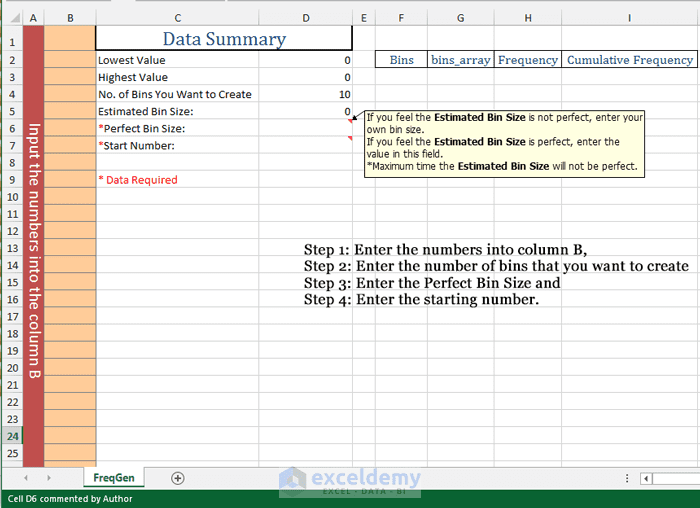FreqGen Excel TemplateYou see from the image, in four steps you can make a frequency distribution table. The steps are:

1. Enter the numbers into column B,
2. Enter the number of bins that you want to create,
3. Enter the perfect bin size and
4. Enter the starting number.

When you will finish inserting all these values, you will get your frequency distribution table on the right side of the template. The table includes:

• The list of bins,
• The bins_array [bins_array is a parameter of Excel FREQUENCY () function],
• The frequencies and
• The cumulative frequencies.

For example, I insert the below 20 numbers into column B, and I enter the bin size as 10.

20, 25, 65, 54, 50, 98, 75, 105, 63, 82, 68, 54, 25, 22, 35, 85, 47, 56, 38, 87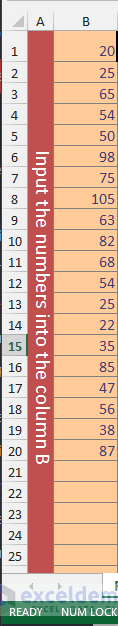Entered the numbers into column B.

Read More: How to Use Sort and Filter with Excel Table

You see in the below image, immediate after I have entered the values into column B, FreqGen template suggests me an Estimated Bin Size as 8. It is not a good idea to take 8 as the bin size. I enter 10 as the Perfect Bin Size and as the starting number I enter 16.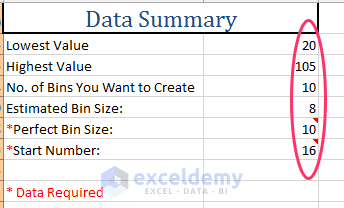Template showing Estimated Bin Size 8. Entered 10 as Perfect Bin Size and as Starting Number I have entered 16.

So you get your Frequency distribution table like the below image. Here goes a little problem. You see the last bin is not necessary for us as it is showing the range from 106 to 115. But our highest value is 105.Unnecessary Last Bin 106-115.

In this type of case, you have to reenter the number of bins you want to create. So I reenter the number of bins as 9. Now you get the perfect result like the image below. Oho!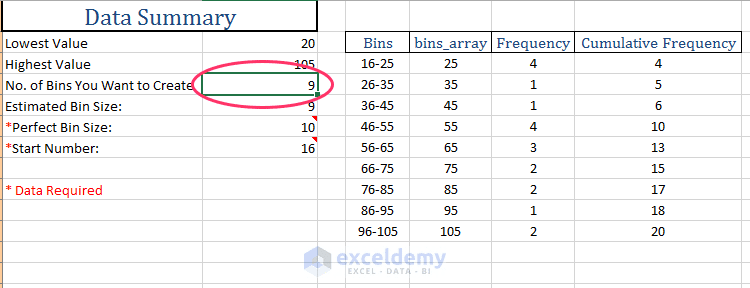I get the perfect Frequency distribution table.

## 2: Frequency Distribution Table Using Pivot Table

But using a pivot table to create an Excel frequency distribution Table is the easiest way.

This part (way 2 of 7) is part of my mastering Excel pivot table series: Pivot Table Tutorials for Dummies: Learn Excel Pivot Table Step by Step

The following figure shows part of a table. The table has a record of 221 students and their test scores. Our goal is to separate the students according to a ten-point range (1–10, 11–20, and so on).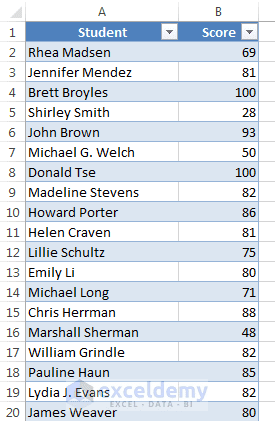We shall separate these students according to ten-point score range.

Read More: How to calculate Average, Median, & Mode in Excel

Creating a pivot table using this table is simple:

### Step 1: Inserting Pivot Table

Select any cell within the table. Click on the Insert tab → In the Tables group of commands choose PivotTable command → Create PivotTable dialog box appears.

In the Create PivotTable dialog box, the table name (in our case it is Table13) is selected under Choose the data that you want to analyze. So our PivotTable will analyze the data of Table13.

Under Choose where you want the PivotTable report to be placed, I select Existing Worksheet and I set Sheet2!\$F\$2 as the Location of the PivotTable report. See the image below: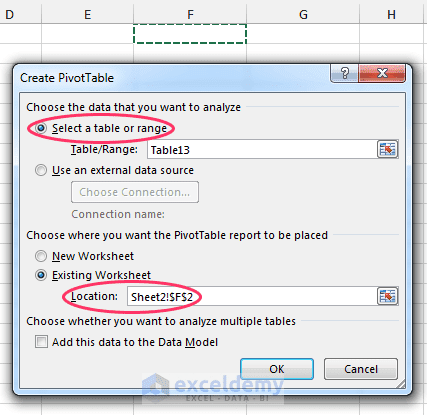Create PivotTable dialog box.

Now click the OK button and you get a pivot table like the below image. On the left, you find the blank pivot table report and on the right, you see the PivotTable Fields task pane (I moved the task pane to place it by the side of the report).Blank PivotTable Report and PivotTable Fields task pane.

PivotTable Fields task pane has two parts: on the left side you will find the fields of the table are listed (in our example only two fields Score and Student) and on the right side the areas where you can drag fields from the left side. There are four areas where you can drag fields from the left side: Filters, Columns, Rows, and Values.

### Step 2: Place the Score field in the Rows area

Place the Score field in the Rows area in the PivotTable Fields task pane. To place a field in an area, you have to take your mouse pointer over the field; the mouse pointer will turn into a four-headed black arrow icon. Now click on your mouse and drag until you reach your area. When you are over the area, just release the mouse.

Or you can right click on a field, and then can choose the area from the drop down.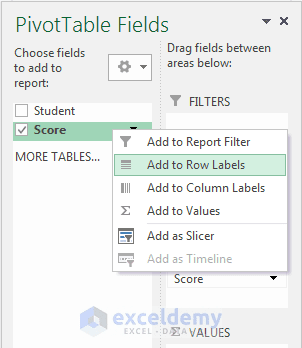Right click on any field and from the drop down choose the area.

### Step 3: Place the Student field in the Values area

In the above-mentioned way, now place the Student field in the Values area. Values of Student field are summarized by counts and you get a pivot table report like the below image: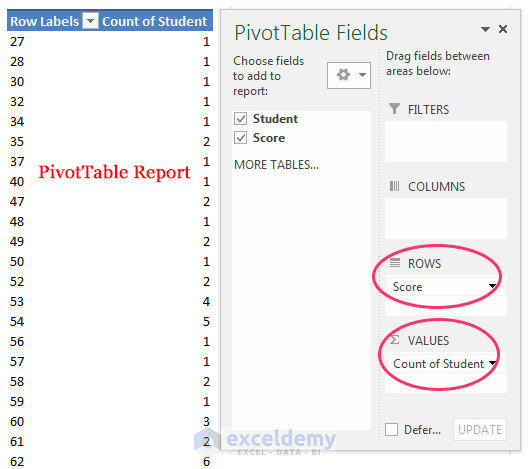Automatically Generated PivotTable Report.

### Step 4: Grouping to get Ten-points Bin or Range

Now we’re going to make a grouping of ten-point range (1–10, 11–20, and so on). Right-click on any value in the Score field column in the pivot table, a shortcut menu will appear. Choose Group from the options of the shortcut menu. Or while selecting a cell under Row Labels column in the PivotTable report, you can click on the ANALYZE contextual tab of PIVOTTABLE TOOLS ⇒ then in the Group group of commands choose the Group Field command. Grouping dialog box will appear.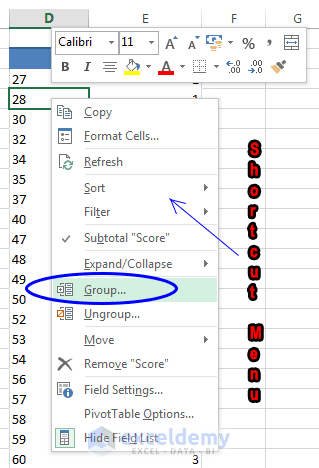Choose Group from this shortcut menu. It appears when you right-click on any value in Score field in the pivot table.

### Step 5: Getting the Grouped Pivot Table

In the Grouping dialog box, you see the Starting at value is 27 as 27 is the lowest value of the score field. I want to make a frequency distribution as 21-30, 31-40, 41-50, and so on. So I enter 21 as the Starting at value. Suggested Ending at value is 100. It is okay. By value is 10 as each bin will have 10 values.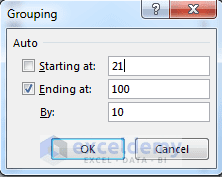Grouping dialog box. I have set 20, 100 and 10 as the Starting at, Ending at and By values respectively.

I click OK button. We shall get a pivot table report like the following figure: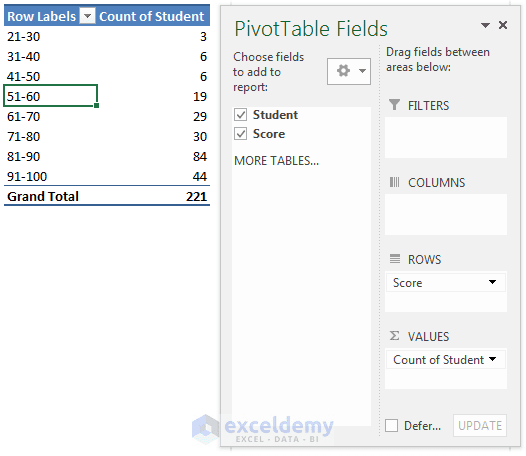The PivotTable report you get after setting the Grouping values.

### Step 6: Showing Items with No Data in the Pivot Table

By default, Excel will not display the values below 21 and above 100 as we have set Starting at value as 21 and Ending at value as 100. But you can force to display the empty bins. To display empty items, you have to right click on any cell under Row Labels and choose Field Settings from the shortcut menu. In the Field Settings dialog box, click the Layout & Print tab, and then select Show Items with No Data. The following figure will make you clear how to display items with no data.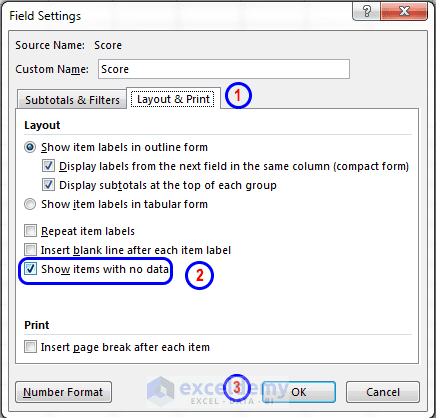Follow these steps to show values  <21 and >100.

But these step is just to show you a technique, you don’t need to show these empty bins when you work with frequency distribution table.

Read More: How to Reference PivotTable Data in Excel Formulas with GETPIVOTDATA?

### Histogram/Frequency Distribution Table & Graph

To get a frequency distribution graph from the above frequency distribution table, at first select any cell within the table. Click on the Insert tab. In the Charts group of commands, you see there is command named PivotChart. Click on the action part of this command (the upper part), Insert Chart dialog box appears with the list of charts that you can create. I select Clustered Column chart and click OK.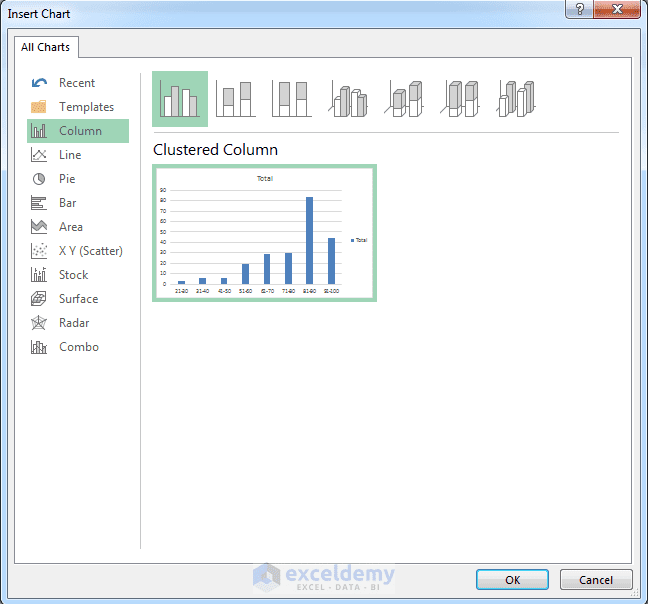I select Clustered Column chart in the Insert Chart dialog box.

When I have clicked the OK button of the Insert Chart dialog box, I have got a chart like the below: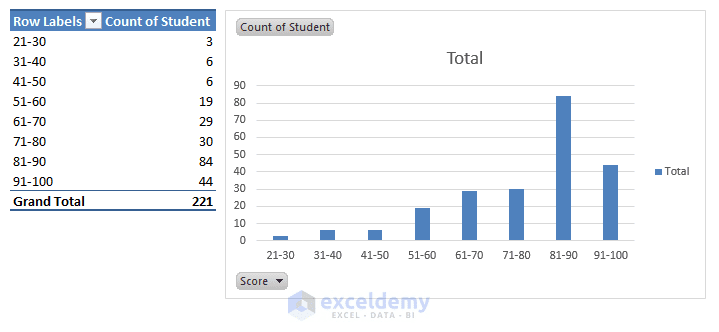The PivotChart I get from the left side PivotTable report.

Note: We have used equal size range (1-10, 11-20, and so on) to create groups automatically in our example. If you don’t want to group the items in equal sized ranges, you can create your own groups. Say, you may want to assign letter grades (A+, A, B, C and so on) based on the students’ score . To do this type of grouping, select the rows for the first group, right-click, and then choose Group from the shortcut menu. Repeat these steps for each new group you want to create. Then change the default group names with more meaningful names. Know more about how to group items in a pivot table.

One hour course on Excel 2016 Pivot Tables (100% Off)

Excel 2016 Pivot Tables: Create Basic Pivot Tables in Excel

## 3. Histogram Using COUNTIFS () function

When COUNTIF () function checks for one criterion, you can use COUNTIFS () function to check multiple criteria.

Syntax of COUNTIF () function: COUNTIF (range, criteria)

Syntax of COUNTIFS () function: COUNTIFS (criteria_range1, criteria1, [criteria_range2, criteria2], [criteria_range3, criteria3]…)

If you don’t know how these two functions work, visit this link for COUNTIF () function and this link for COUNTIFS () function.

To show you how to make a frequency distribution table in Excel using COUNTIFS () function I am going to use three examples.

Say your company surveyed 100 people to know two things:

1. How many children each of the surveyees have
2. And their yearly income.

You get a result like the following image: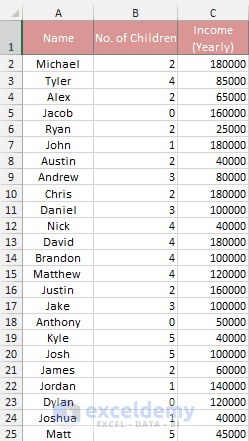Part of the Survey Data

Your boss ordered you to make two frequency distribution tables: one for No. of Children and another one for Income (Yearly).

Before making frequency distribution, let’s give the ranges some unique names.

• The No. of Children range is B2: B101, I will name it as Children.
• And the Yearly Income range is C2: C101, I will name it as Income.

If you don’t know how to create a named range, follow me:

You can name a range in many ways. I am going to use one of them.

• Click on the Formulas tab → In the Defined Names group, click on the Name Manager Command → Name Manager Dialog box appears.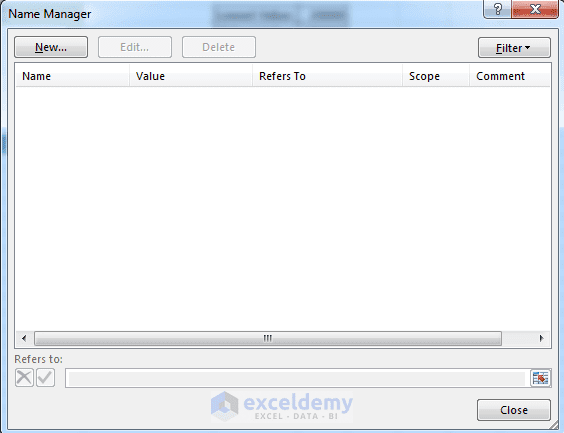Blank Name Manager Dialog box.

• You see Name Manager Dialog box is blank. It means you did not create any named range in your workbook. Click on the New. New Name Dialog box appears. In the Name field, I type Children. As Scope workbook is selected. Scope workbook means you can use the name range Children from the whole workbook. In the Comment box, I don’t input anything. So it is blank. In the Refers to field, I type =Survey!\$B\$2:\$B\$101.New Name dialog box. I have entered values into the fields.

• If you click OK, then cell range \$B\$2:\$B\$101 will be named as Children. In the same way, I name range \$C\$2:\$C\$101 as Income. So the Name Manager Dialog box will now have two names: Children and Income.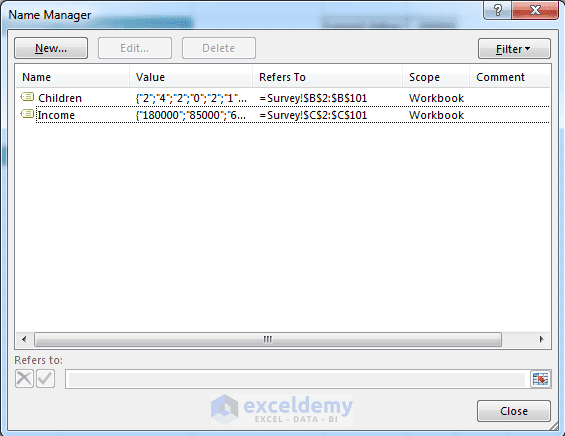Now Name Manager Dialog box has two names: Children and Income.

### Example #1 – Let’s find out the frequency distribution of No. Of Children Column

At first, I find out the lowest value and highest value of No. Of Children column. They are 0 and 5 respectively. To find out the lowest and highest value, use MIN () and MAX () functions respectively.

So for column No. of Children, there is no use of making a frequency distribution like 0-1, 2-3 and 4-5. I use straight 0, 1, 2, 3, 4, and 5 and use the following formula in cell I3: =COUNTIFS (Children, “=”&H3)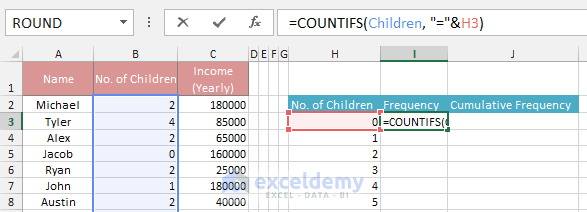Formula in cell I3: =COUNTIFS(Children, “=”&H3)

I copy the formula from cell I3 to other cells (I4: I8). So you get frequency distribution like the below image: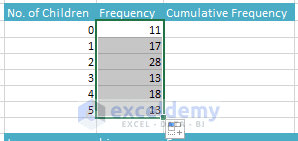Frequency Distribution Table that I get from No. of Children column.

### Get the Cumulative Frequency Distribution

On cell J3 I input formula: =I3

On cell J4 I input formula: =J3+I4

Now copy the formula from cell J4 to other cells below (J5: J8). So you get a cumulative frequency distribution table like below: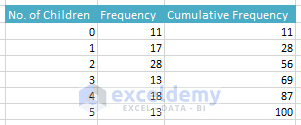Finally, I get both Frequency Distribution Table & Cumulative Frequency Distribution Table.

### Example #2 – Now let’s find out the frequency distribution of Income (Yearly) Column

The lowest and highest values of Income column is 20,000 and 180,000 respectively. Say you want to make a frequency distribution using the following bins:

1. 50000 or less
2. 50001 – 70000
3. 70001 – 90000
4. 90001 – 110000
5. 110001 – 130000
6. 130001 – 150000
7. Over 150001

I input the above bins manually like the image below. I also define the bins_array values (you know the highest values of the bins make the bins_array. In the image, you see: the last bin has no highest value so the bins_array value for this bin is blank).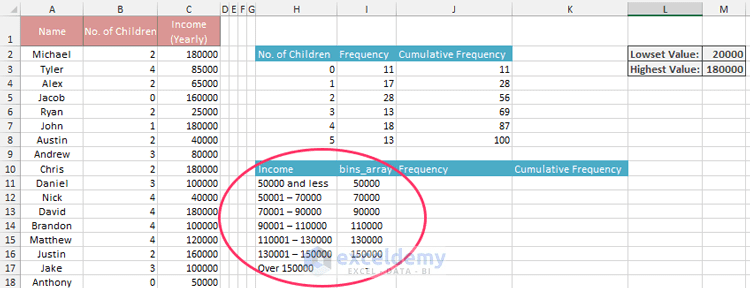Manually created bins and bins_array from Income (Yearly) column.

Of 7 bins, the first bin and last bin are of different size. Other bins from 2nd to 6th are of the same size. So we have to write different formulas for the first and last bin and one formula for other bins (from 2nd to 6th bin).

For the first bin, I write a formula in cell J11 as =COUNTIFS (Income, “<=”&I11)Formula in cell J11: =COUNTIFS(Income, “<=”&I11)

In the same way, for the last bin, I write a formula in cell J17 as =COUNTIFS (Income,”>150000″)

In cell J12 I write a formula as: =COUNTIFS (Income, “>”&I11, Income, “<=”&I12)

And I copy this formula (formula in cell J12) from cell J13 to J16. Now you get both regular and cumulative frequency distribution (I set the formula already) table like the below image.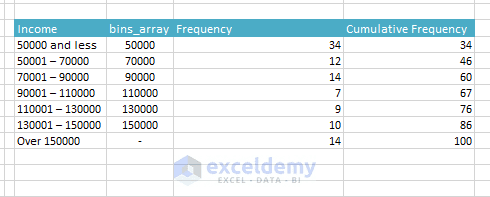Frequency Distribution Table and Cumulative Frequency Distribution Table.

So you are done.

### Example #3 – Now we shall create a frequency distribution from some text

Look at the following example. The Names column has total 50 names. Our first job is to list the unique names in a separate column. Next job is to find out the occurrences (frequencies) of the names in the column.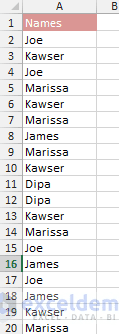Part of Names column.

You can use the Advanced filter command in the Data ribbon to list the unique names in a separate column. If you don’t know how to do that, follow me:

• Click on the Data tab. In the Sort & Filter group of commands click on the Advanced command. Advanced Filter dialog box appears.
• Under Action you will find two options: Filter the list, in-place, and Copy to another location. I select Copy to another location radio button. In the List range field, I input range \$A\$1: \$A\$51 (including the column heading Names).
• I will let Criteria range as blank. In the Copy to field, I input \$C\$2.
• And finally, I select the check box Unique records only. After doing all the above things, you will get Advanced Filter dialog box like the image below: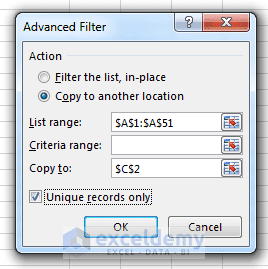• Now click OK You will get a list of unique records in cell C2 like the image below.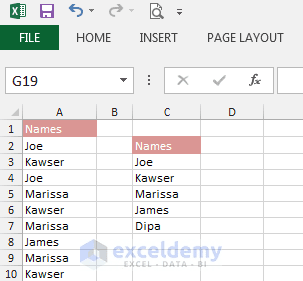Unique names filtered in different cells.

Now let’s find out the Frequency and Cumulative Frequency of these names

Now it is very simple to find out the frequency of these names. On cell D2 and E2, I input Frequency and Cumulative Frequency respectively.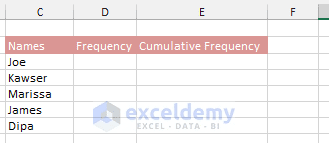Frequency Distribution Table.

On cell D3 I input this formula: =COUNTIF (\$A\$2: \$A\$51, C3)

Then I copy this formula for other cells below. At the same time, I get the Cumulative Frequency Distributions [Image Below].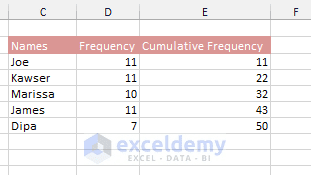Frequency Distribution Table.

## 4. Histogram with Frequency () Function

Let’s show you how to use FREQUENCY () function to make frequency distribution in Excel. To narrate the process I shall use the survey data again that I have used in way 3 of 7 part of this tutorial. If you forgot I want to remind you here again: your company surveyed 100 people to know their no. of children and yearly income and got data like the image below.I have named No. of Children column as Children and Income (Yearly) column as Income. In this part of the tutorial, I shall calculate the frequency distribution of Income (Yearly) column. The lowest and highest values of Income (Yearly) column are 20,000 and 180,000 respectively. And I want to use the bins_array like the image below.Bins and bins_array created from the Income (Yearly) column.

Before going into deep, at first let’s introduce you with the FREQUENCY () function of Excel.

Take a look at the syntax of FREQUENCY () function: FREQUENCY (data_array, bins_array)

FREQUENCY () function has two arguments:

1. data_array: it might be an array or a range of cells for which you want to make frequency distribution. As we are going to find out the frequency distribution of Income (Yearly) column and we have named the data of this column as Income so the value of the data_array argument is Income.
2. bins_array: these are the intervals into which you want to group of the values of data_array. You already know that the higher values of the intervals make the bins_array. In our example, bins_array is already created as shown in above image.

Steps to create frequency distribution:

1. Select cell J3 to J9 in the survey worksheet.
2. Now create this formula in the formula bar or in cell J3: =FREQUENCY (Income, \$I\$3:\$I\$8)
3. Don’t press the ENTER key on your keyboard. I have selected total 7 cells (from cell J3 to J9) to input the above formula because I want to create an array formula.
4. To enter the formula as an array formula into cells J3 to J9, press CTRL + SHIFT + ENTER key simultaneously on the keyboard.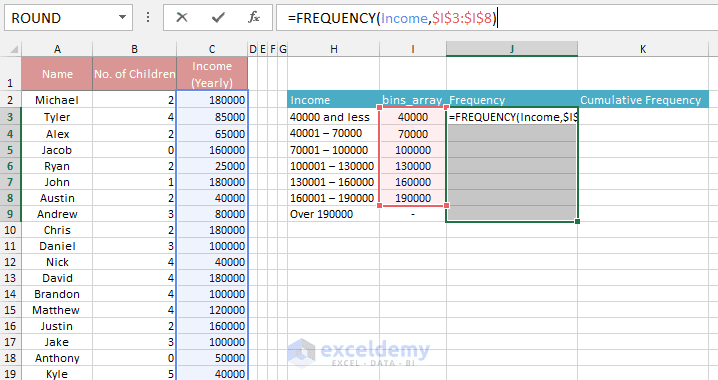Formula entered into cell J3.

5. You will get the following frequency distribution table. You will also get cumulative frequency distribution table on the right side of the table.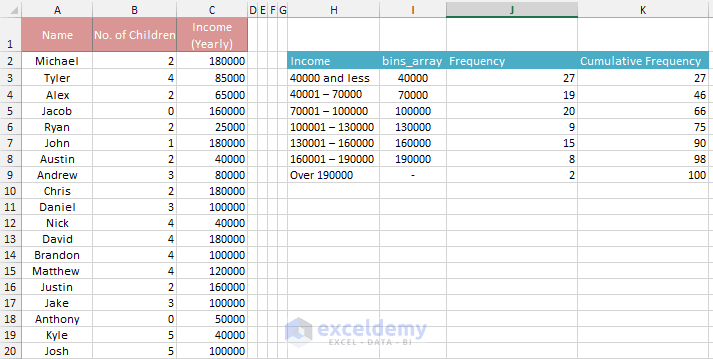Frequency and Cumulative Frequency Distribution Table

So you are done. So simple 🙂

## 5. Frequency distribution with Frequency () & Index () functions

I learned this technique from Charley Kyd of ExcelUser.com. I will use Excel’s Frequency () and Index () functions. However, to understand this process, know very well:

1. How Excel’s array formula works. Introduce yourself with Excel’s array formula using this link.
2. And how Excel’s Index () function works. Use this Microsoft office tutorial to learn about Index () function.

To use this method in creating frequency distribution, I have used again the survey data and I shall make a frequency distribution of Income (Yearly) column. In this method, I have to input an extra column into the frequency distribution table as you see in the image below (I have put it on the left of the table). The column (in the image the ‘#’ column) will show the serial number of the frequency distribution row.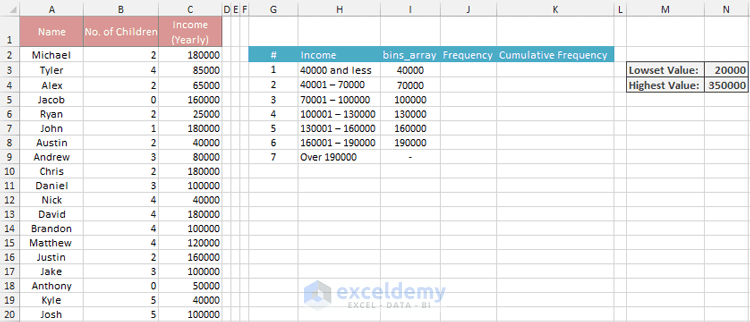You see the new # column on the left side of the table.

Excel’s Frequency () function internally generates an array. In cell J3, I enter this formula:

=FREQUENCY (Income, \$I\$3:\$I\$8)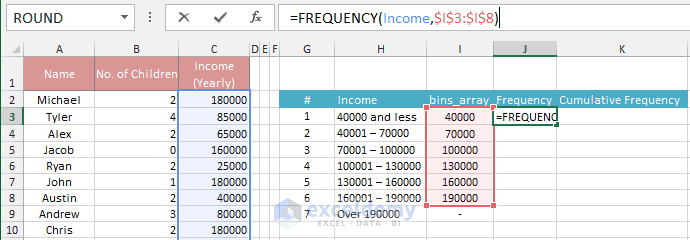In cell J3, I have entered this formula: =FREQUENCY (Income, \$I\$3:\$I\$8)

You know to enter a formula as an array formula, you have to press CTRL + SHIFT + ENTER keys in the keyboard. But in this case, I don’t want to create an array formula. I just want to show you how the Frequency () function generates an array internally. I just press Enter and the cell J3 shows value 27.

Now select cell J3 again and click anywhere on the formula in the formula bar. Now press the F9 key in the keyboard. While your cursor is in the formula bar and within a formula and you press the F9 key, the formula bar shows the value of the formula. You see an array as the image below: = {27; 19; 20; 9; 15; 8; 2}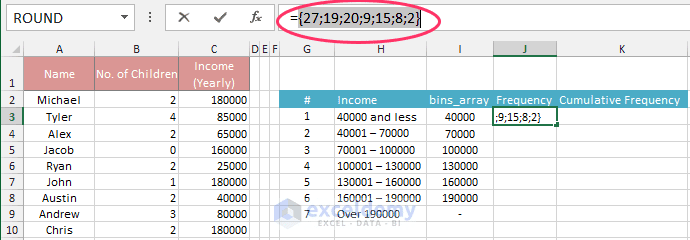Internally created array by the Frequency () function.

The values in an array can be semi-colon separated or comma separated. When the values are semi-colon separated, their orientation in Excel sheet will be Vertical and when the values are comma separated, their orientation will be Horizontal.

So the cell J3 is showing the value 27 (the first value of the array) but the formula is internally holding an array actually. The 1st value of the array is 27, the 2nd value of the array is 19 and so on.

So how do we use Index () function? Rewrite the formula in cell J3 in this way:

=INDEX (FREQUENCY (Income, \$I\$3:\$I\$8), G3)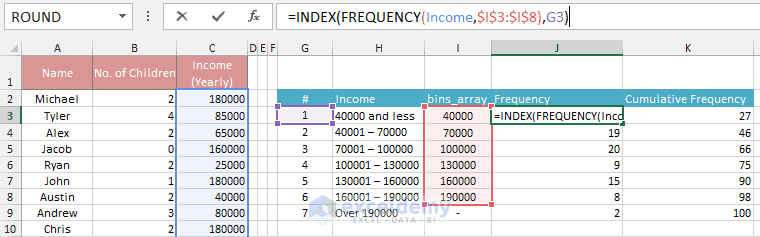Modified Formula in cell J3.

Copy paste the formula from cell J3 to J9. And you get your frequency distribution table with the cumulative frequency distribution.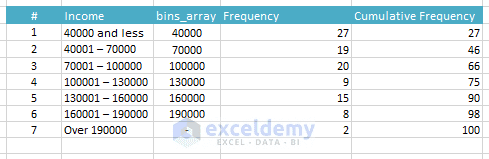You get this Frequency Distribution Table finally.

So, you are done. You can hide the # column if you wish 🙂

## 6. Histogram with SUM () and IF () Functions

To understand this process you have to know very well:

1. How Array Formula works. Know about array formula using this link.
2. How SUM () function works. Know about SUM () Function using this link.
3. And how IF () function works. Know about IF () Function using this link.

Again we shall use the Income (Yearly) column of survey worksheet and the following bins to make a frequency distribution.Bins and bins_array created from the Income (Yearly) column.

First and last bin are of different sizes. So we have to create a different formula for these two bins and the same formula for other bins (2nd to 6th bins). 2nd to 6th bins are of the same size.

For the first bin, I create this formula in cell J3: =SUM (IF (Income<=I3, 1, 0))

For the 2nd to 6th bins I select cells from J4 to J6 and create this formula:

=SUM (IF ((Income>I3)*(Income<=I4), 1, 0))

And for the last bin I create this formula in cell J7: =SUM (IF ((Income>I8), 1, 0))

Remember that you have to enter all those three formulas as array formulas. Press CTRL + SHIFT + ENTER (CSE) keys on your keyboard to enter those formulas as array formulas.

And you get your frequency distribution.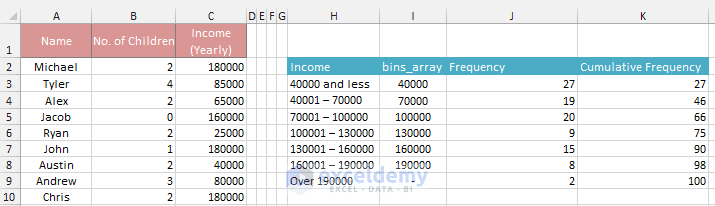Frequency Distribution Table – Final Result.

Let’s explain the formula of cell J5 and you will be able to understand the rest of the formulas.

The formula in cell J5 is: =SUM (IF ((Income>I4)*(Income<=I5), 1, 0))

Income is the name range of cells: C2: C101. Values of cells I4 and I5 are 70000 and 100000 respectively. So we can rewrite the formula like below:

=SUM (IF ((C2: C101>70000)*(C2: C101<=100000), 1, 0))

According to array formula rules, internally IF function part of this formula will be expanded by Excel in the following ways:

1. IF ((C2>70000)*(C2<=100000), 1, 0); it returns 0.
2. IF ((C3>70000)*(C3<=100000), 1, 0); it returns 1.
3. IF ((C4>70000)*(C4<=100000), 1, 0); it returns 0.
4. IF ((C5>70000)*(C5<=100000), 1, 0); it returns 0.
5. … … … … … … … … … … … … … … … …
6. … … … … … … … … … … … … … … … …
7. … … … … … … … … … … … … … … … …
8. IF ((C100>70000)*(C100<=100000), 1, 0); it returns 1.
9. IF ((C101>70000)*(C101<=100000), 1, 0); it returns 0.

So the whole formula becomes like this: =SUM ({0, 1, 0, 0, …, …, …, 1, 0}). And the sum of this array 20 is showed in cell J5.

You are done!

Related: Stock Return Analysis Using Histograms & 4 Skewness Of Histograms

## 7. Using SUMPRODUCT () Function to make a histogram

The last way to make frequency distribution table in Excel is using SUMPRODUCT () Function.

I have used the same survey data, the same Income (Yearly) column and the same bins_array to make the frequency distribution table. Take a look at the following image:Bins and bins_array created from the Income (Yearly) column.

Syntax of SUMPRODUCT () function: SUMPRODUCT (array1, [array2], [array3], [array4] …)

Observe the syntax of the SUMPRODUCT () function. As the arguments of SUMPRODUCT () function, you can pass arrays or ranges. If you pass a range, the cell range will be treated as an array. And you know you can perform operations on arrays.

Some examples of performing operations on arrays:

1. {1, 2, 3}*2 will generate a new array: {1*2, 2*2, 3*2} = {2, 4, 6}
2. {1, 2, 3}+2 will generate a new array: {1+2, 2+2, 3+2} = {3, 4, 5}
3. {TRUE, FALSE, TRUE, TRUE, FALSE}+1 = {TRUE + 1, FALSE + 1, TRUE + 1, TRUE + 1, FALSE + 1} = {2, 1, 2, 2, 1}; TRUE is treated as 1 and FALSE is treated as 0.
4. {TRUE, FALSE, TRUE, TRUE, FALSE}*1 = {TRUE*1, FALSE*1, TRUE*1, TRUE*1, FALSE*1} = {1, 0, 1, 1, 0}
5. {110, 20, 50}>=100 will generate a new array as {110>=100, 20>=100, 50>=100} = {TRUE, FALSE, FALSE}

Okay, now let’s calculate the frequency distributions using the SUMPRODUCT () function.

For the first bin and last bin I have to build two different formulas and for the other bins (2nd bin to 6th bin), I will build one formula and then will copy that formula to other cells.

For the first bin, in cell J3 I write this formula: =SUMPRODUCT (– (Income<=I3))

For the last bin, in cell J9 I write this formula: =SUMPRODUCT (– (Income>I8))

And for the other bins, in cell J4 I write this formula: =SUMPRODUCT ((Income>I3)*(Income<=I4))

Now let’s explain how the formula in cell J3 works: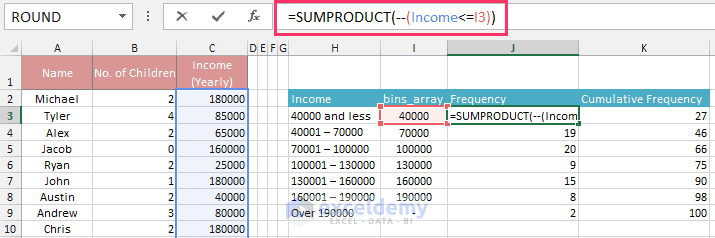The formula in cell J3.

You see in the formula SUMPRODUCT () function uses only one array (array1). The array is: Income; but the array is filtered with an operation: <=I3. Value of I3 is 40,000. Let’s see the step by step internal calculations:

For value of cell C2: –(Income < = I3) becomes: –(180000 <= 40000) = –FALSE = 0

For value of cell C3: –(Income < = I3) becomes: –(85000 <= 40000) = –FALSE = 0

For value of cell C4: –(Income < = I3) becomes: –(65000 <= 40000) = –FALSE = 0

For value of cell C5: –(Income < = I3) becomes: –(160000 <= 40000) = –FALSE = 0

For value of cell C5: –(Income < = I3) becomes: –(25000 <= 40000) = –TRUE = 1

… … … … … … … … … … … … … … … … … … … … … … … …

… … … … … … … … … … … … … … … … … … … … … … … …

In this way: at the end of calculations, you will get an array as: {0; 0; 0; 0; 1; …}

And when SUMPRODUCT () function is applied on a single array, it shows the sum of that array. In this way, the cell J3 shows 27. I think now you will be able to explain how the other formulas work.

oho! You are done with all the 7 ways.

## Wrapping Up

I have shared all the seven ways here. If you know any other way to make frequency distribution table in Excel or any question arises in your mind about the above discussion, put it in the comments section.
Happy Excelling 🙂

How to Make a Histogram in Excel Using Data Analysis ToolPak

How to Calculate/Find Mean and Standard Deviation in Excel

How to Calculate Variance in Excel

Frequency-distribution1.xlsx

Frequency-Distribution-Table-Excel.xlsx

Frequency-Distribution-Table-Excel-Way-4.xlsx

Frequency-Distribution-Table-Excel-Way-5.xlsx

Frequency-Distribution-Table-Excel-Way-6.xlsx

Frequency-Distribution-Table-Excel-Way-7.xlsx

Hello!
Welcome to my Excel blog! It took me some time to be a fan of Excel. But now I am a die-hard fan of MS Excel. I learn new ways of doing things with Excel and share here. Not only how to guide on Excel, but you will get also topics on Finance, Statistics, Data Analysis, and BI. Stay tuned!

1. Replyraj Oct 28, 2015 at 4:21 PM

20 30 45 66 35
34 55 27 44 66
44 23 78 45 77
55 45 87 98 97

ques? how to insert all these values into one column in excel

2. ReplySep 27, 2016 at 11:46 PM

Thank you this is wonderfull